# Count Sort : Explained with Examples

Problem Statement: Given an array sort the elements of the array using count sort.

### Introduction to Count Sort

Count sort is one of the non-comparison stable sorting algorithms which sorts elements within a range k in O(n+k) time complexity.

### Properties of Count Sort

1. It is a linear time algorithm when input lies within a small range k i.e. k should be linear with respect to n.
2. It is a non-comparison based sorting algorithm because it counts the occurrences of elements and on that basis, sorting takes place.
3. It is a stable sorting algorithm.
4. It is used as a subroutine in radix sort.

### Solution :

Disclaimer: Don’t jump directly to the solution, try it out yourself first.

### Approach 1: Naive Approach

Count sort counts the occurrences of elements and later places them into the array with respect to the number of occurrences. The following steps explain this approach.

1. We create a count array of size k and initially define every index with a value of 0. Since elements lie within a range k and we need to count the occurrence of those elements, we store the occurrence of that number at the respective index.
2. For every number in that range, we run a loop for the number of times that number occurred in the array and continue to place that number in the array for that number of times.
3. We end up with the final sorted array.

Let’s visualize this approach using an example and diagram

Let arr[]={5, 6, 5, 2}, k=7, n= 4

Using step 1, our count array would be as follows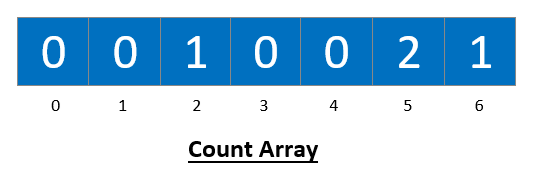Now for every number between 0 and k-1, both inclusive we place that number in our original array from 0 to n-1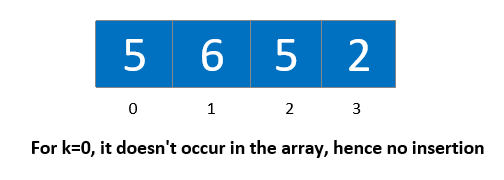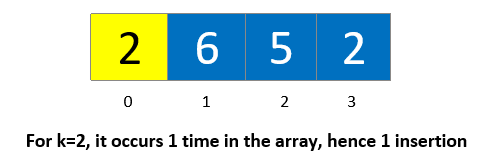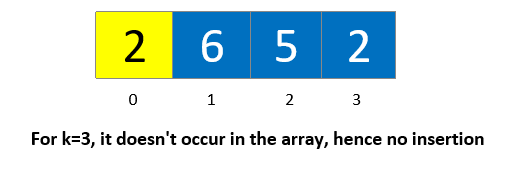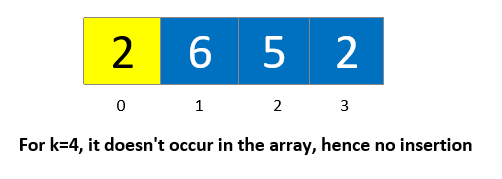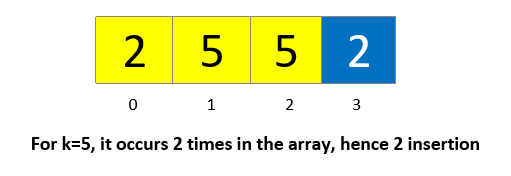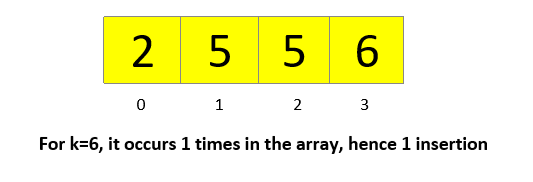Code:

## C++ Code

``````#include <iostream>
using namespace std;

void countSortNaive(int arr[], int k, int n) {
int count[k];
for (int i = 0; i < k; i++) {
count[i] = 0;
}
for (int i = 0; i < n; i++) {
count[arr[i]]++;
}
int index = 0;
for (int i = 0; i < k; i++) {
for (int j = 0; j < count[i]; j++) {
arr[index] = i;
index++;
}
}
for (int i = 0; i < n; i++) {
cout << arr[i] << " ";
}
}
int main() {
int n = 4, k = 7;
int arr[] = { 5, 6, 5, 2 };
countSortNaive(arr, k, n);
return 0;
}
``````

Output: 2 5 5 6

Time Complexity: O(n*k)

Space Complexity: O(k)

## Java Code

``````public class Main {
static void countSortNaive(int arr[], int k, int n) {
int[] count = new int[k];
for (int i = 0; i < k; i++) {
count[i] = 0;
}
for (int i = 0; i < n; i++) {
count[arr[i]]++;
}
int index = 0;
for (int i = 0; i < k; i++) {
for (int j = 0; j < count[i]; j++) {
arr[index] = i;
index++;
}
}
for (int i = 0; i < n; i++) {
System.out.print(arr[i] + " ");
}
}
public static void main(String args[]) {
int arr[] = { 5, 6, 5, 2};
int n = 4;
int k = 7;
countSortNaive(arr, k, n);
}
}
``````

Output: 2 5 5 6

Time Complexity: O(n*k)

Space Complexity: O(k)

### Approach 2: Efficient Approach

With some additions and modifications to the naive approach, we can create a general-purpose algorithm. Let’s see how we can do it.

1. We create a count array of size k and initially define every index with a value of 0. Since elements lie within a range k and we need to count the occurrence of those elements, we store the occurrence of that number at the respective index.
2. We modify our count array – for every i varying from 0 to k-1, we store the number of elements smaller than or equal to the i.
3. Now we build our resultant array
1. We traverse the original array from the end.
2. We use these elements in the array as the index of our count array to determine the number of elements (a) smaller or equal to that element.
3. Once we get that number(a), we place that element at that ath position i.e. [a-1]th index in the resultant array.
4. Once we place it at that position, we reduce a.

Finally, we copy the contents of our resultant array to the original array.

Note: We are performing backward iteration in 3. a to keep the algorithm stable.

Let’s understand this using diagrams

`Let arr[]={5, 6, 5, 2}, k=7, n= 4`

Using step 1, our count array would be as followsAfter modification, our new count array would look as follows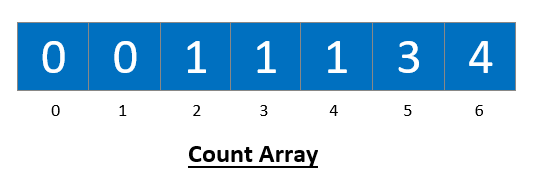It’s time to build the res array. We perform backward iteration in the original array and use that number as an index in the modified count array to get the amount of numbers (a) greater than that element. Later we insert that number at [a-1]th index in the res array and we reduce a by 1.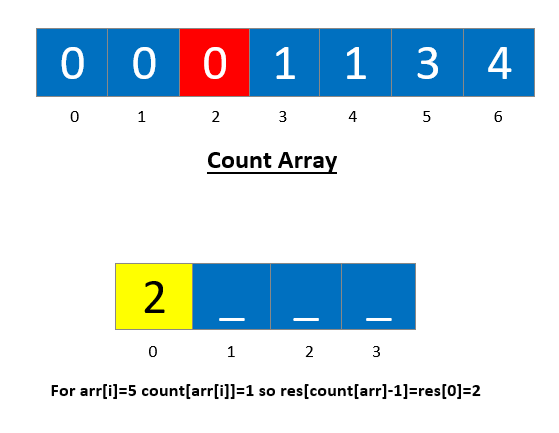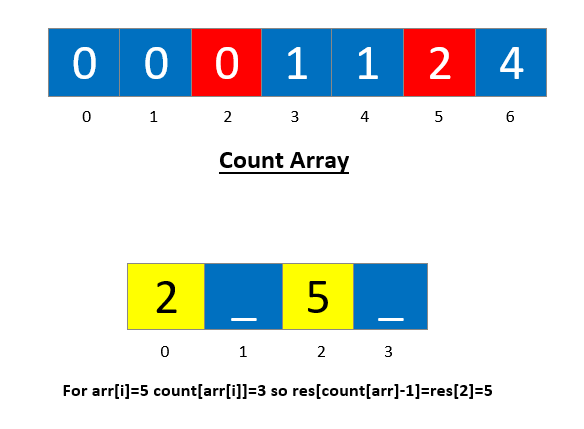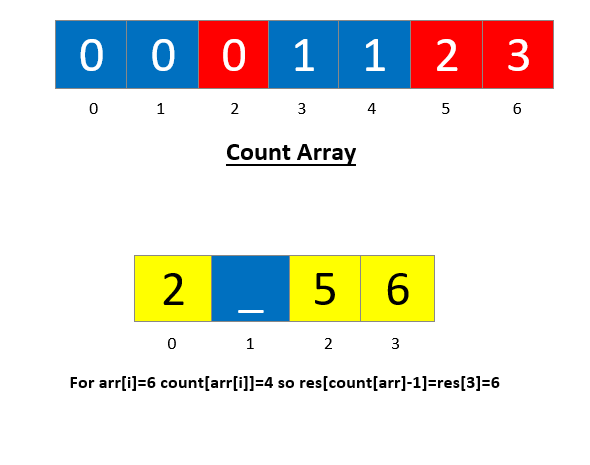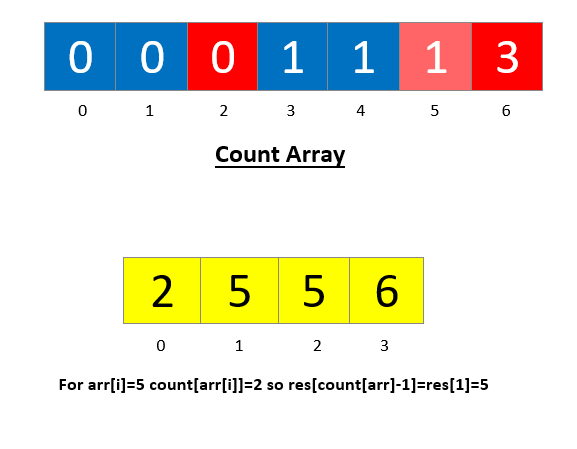Code:

## C++ Code

``````#include <iostream>
using namespace std;

void countSortEfficient(int arr[], int k, int n) {
int count[k];
for (int i = 0; i < k; i++) {
count[i] = 0;
}
for (int i = 0; i < n; i++) {
count[arr[i]]++;
}
for (int i = 1; i < k; i++) {
count[i] = count[i - 1] + count[i];
}
int res[n];
for (int i = n - 1; i >= 0; i--) {
res[count[arr[i]] - 1] = arr[i];
count[arr[i]]--;
}
for (int i = 0; i < n; i++) {
arr[i] = res[i];
cout << arr[i] << " ";
}
}
int main() {
int n = 4, k = 7;
int arr[] = { 5, 6, 5, 2};
countSortEfficient(arr, k, n);
return 0;
}
``````

Output: 2 5 5 6

Time Complexity: O(n+k)

Space Complexity: O(n+k)

## Java Code

``````public class Main {
static void countSortNaive(int arr[], int k, int n) {
int[] count = new int[k];
for (int i = 0; i < k; i++) {
count[i] = 0;
}
for (int i = 0; i < n; i++) {
count[arr[i]]++;
}
int index = 0;
for (int i = 0; i < k; i++) {
for (int j = 0; j < count[i]; j++) {
arr[index] = i;
index++;
}
}
for (int i = 0; i < n; i++) {
System.out.print(arr[i] + " ");
}
}
static void countSortEfficient(int arr[], int k, int n) {
int[] count = new int[k];
for (int i = 0; i < k; i++) {
count[i] = 0;
}
for (int i = 0; i < n; i++) {
count[arr[i]]++;
}
for (int i = 1; i < k; i++) {
count[i] = count[i - 1] + count[i];
}
int[] res = new int[n];
for (int i = n - 1; i >= 0; i--) {
res[count[arr[i]] - 1] = arr[i];
count[arr[i]]--;
}
for (int i = 0; i < n; i++) {
arr[i] = res[i];
System.out.print(arr[i] + " ");
}
}
public static void main(String args[]) {
int arr[] = { 5, 6, 5, 2 };
int n = 4;
int k = 7;
countSortEfficient(arr, k, n);
}
}
``````

Output: 2 5 5 6

Time Complexity: O(n+k)

Space Complexity: O(n+k)

Special thanks to Yash Mishra for contributing to this article on takeUforward. If you also wish to share your knowledge with the takeUforward fam, please check out this article